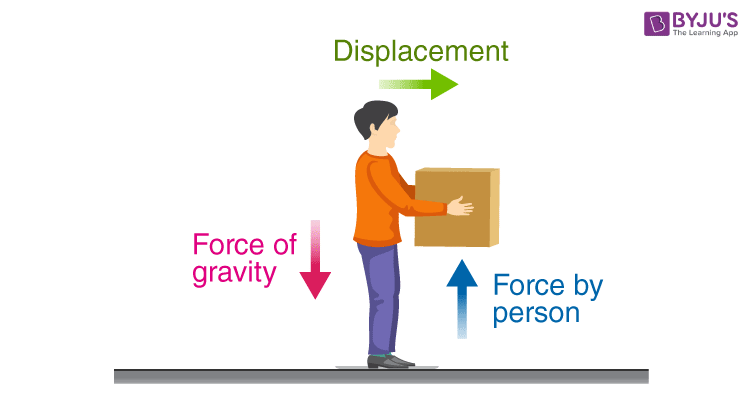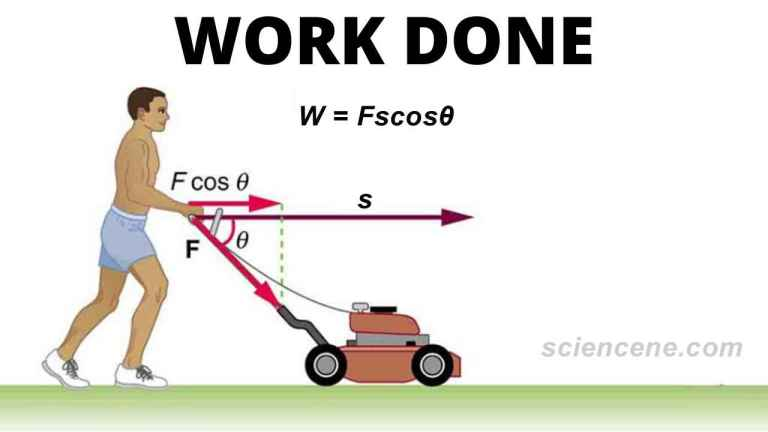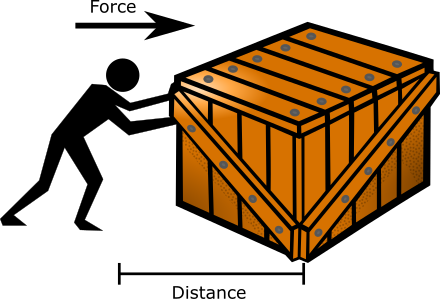Swati Talwar

HS-PS3-1

# Work Study Guide

In this chapter, we explore the concept of work in detail. When is work said to be done and when can work be negative? It is all in the mathematical formula of the concept.

Table of content

## INTRODUCTIONSource

The strike of a hammer drives a nail into a piece of wood; by exerting an upward force on the handle, we are able to lift a bucket filled with sand off the ground. In both instances, we are applying energy to get work done. The concept of work is central to physics and understanding the interaction of matter and energy, so let’s get started!

## WHAT IS WORK?Source

Although the word work has different meanings in everyday usage, the definition of work in physics is more precise. The work done is measured as a product of the force applied on an object and the distance it moves because of the force. Mathematically that translates to:

W = Fs

Here,

W is the work done measured in joules (J), the unit of energy

F is the force applied on the object in newtons (N)

And s is the distance moved by the object in meters (m)

If the force is acting at an angle (θ) to the displacement, then it can also be written as

W = Fs cos θ

## FACTORS AFFECTING WORKSource

Going by the formula calculating work done, we can see that there are two things that affect work:

## FORCE

• Force is an agency that can push or pull an object to make it move or change the direction of motion of a moving object.
• Force is also needed to bring a moving object to rest and to change the shape of an object.
• Let’s say you’re lifting a bucket filled with water.
• To get the bucket off the ground, you will have to apply a force that is equal to the weight of the bucket.

## DISPLACEMENT

When you continue lifting the bucket higher, you are doing more work by displacing the bucket from its original position. Let’s say the bucket weighs 100 Newton, and you lift it up by 1 meter. Your work done can be calculated as:

W = Fs = (100 N) (1 m) = 100 Nm = 100 joules

If you lift it up by 2 meters, then the work done is,

W = Fs = (100 N) (2 m) = 200 Nm = 200 joules

If the displacement is zero, then the work done is also zero. If you are unable to lift the bucket, then the work done is zero!

## SUMMARY

• Work is said to be done when the force applied to the object makes it move.
• Mathematically work done is defined as W = Fs.
• If the displacement of the object is zero, then the work done is zero.

## FAQs

Q. What is the standard unit of measurement for work?

The SI unit of workis the Joule (J). It can also be expressed as a Newton-meter (N-m).

Q. When a body slides against a rough surface, the work done by friction is positive or negative?

Both force and displacement that define work done are vector quantities. Therefore if the force acting on the rough surface is in the opposite direction to the displacement, then the frictional work done is negative.

Q. What is the rule of work in physics?

Mathematically, work done is W = Fs. However, if the force is acting at an angle (θ) to the displacement, then it can also be written as     W = Fs cos θ

Q. Can work be negative?

Yes, if the force is acting in the direction opposite to the displacement of the body, the work can be said to be negative.

We hope you enjoyed studying this lesson and learned something cool about Work! Join our Discord community to get any questions you may have answered and to engage with other students just like you! Don't forget to download our App to experience our fun VR classrooms - we promise, it makes studying much more fun! 😎

1. Work:https://flexbooks.ck12.org/cbook/ck-12-physics-flexbook-2.0/section/7.1/primary/lesson/work-phys/ Accessed 11th April 2022
2. What is Work? https://www.khanacademy.org/science/physics/work-and-energy/work-and-energy-tutorial/a/what-is-work Accessed 11th April 2022
3. Work Done: https://byjus.com/physics/work-done/ Accessed 11th April 2022
4. Work: https://byjus.com/physics/work/ Accessed 11th April 2022